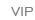# 名师作品

•[db:格式]

•[db:格式]

•[db:格式]

•[db:格式]

•[db:格式]

•[db:格式]

•[db:格式]

•[db:格式]

•[db:格式]

•[db:格式]

•[db:格式]

•[db:格式]

#### 有机农场logo图片

•[db:格式]

•[db:格式]

•[db:格式]

•[db:格式]

•[db:格式]

•[db:格式]

•[db:格式]

•[db:格式]

•[db:格式]

•[db:格式]

•[db:格式]

•[db:格式]

•[db:格式]

•[db:格式]

•[db:格式]

•[db:格式]

•[db:格式]

•[db:格式]

•[db:格式]

•[db:格式]

•[db:格式]

•[db:格式]

•[db:格式]

•[db:格式]

•[db:格式]

•[db:格式]

••